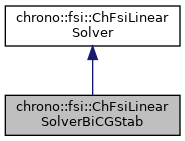chrono::fsi::ChFsiLinearSolverBiCGStab Class Reference

## Description

GPU implementation of BICGSTAB method via CUDA libraries.

#include <ChFsiLinearSolverBiCGStab.h>

Inheritance diagram for chrono::fsi::ChFsiLinearSolverBiCGStab:[legend]
Collaboration diagram for chrono::fsi::ChFsiLinearSolverBiCGStab:[legend]

## Public Member Functions

ChFsiLinearSolverBiCGStab (double mrel_res=1e-8, double mabs_res=1e-4, int mmax_iter=1000, bool mverbose=false)

virtual SolverType GetType () override
returns the type of the linear solver

virtual void Solve (int SIZE, int NNZ, double *A, unsigned int *ArowIdx, unsigned int *AcolIdx, double *x, double *b) override
implements the abstract method of the base class using BICGSTAB More...Public Member Functions inherited from chrono::fsi::ChFsiLinearSolver
ChFsiLinearSolver (double mrel_res=1e-8, double mabs_res=1e-4, int mmax_iter=1000, bool mverbose=false)
Constructor of the ChFsiLinearSolver. More...

void SetVerbose (bool mv)
Set verbose output from solver.

bool GetVerbose () const
Return whether or not verbose output is enabled.

double GetResidual ()
Return the current residual.

int GetNumIterations ()
Return the number of current Iterations.

void SetIterationLimit (int numIter)
Set and Get for the max_iter.

int GetIterationLimit ()

void SetAbsRes (double mabs_res)
Set and Get for the abs_res and.

double GetAbsRes ()

void SetRelRes (double mrel_res)

double GetRelRes ()

int GetSolverStatus ()
Return the solver status 0: unsuccessful 1: successfully converged.Public Types inherited from chrono::fsi::ChFsiLinearSolver
enum  SolverType {
BICGSTAB = 0, GMRES, CR, CG,
SAP
}Protected Attributes inherited from chrono::fsi::ChFsiLinearSolver
double rel_res = 1e-3

double abs_res = 1e-6

int max_iter = 500

bool verbose = false

int Iterations = 0

double residual = 1e5

int solver_status = 0

## ◆ ChFsiLinearSolverBiCGStab()

 chrono::fsi::ChFsiLinearSolverBiCGStab::ChFsiLinearSolverBiCGStab ( double mrel_res = 1e-8, double mabs_res = 1e-4, int mmax_iter = 1000, bool mverbose = false )
inline
Parameters
 mrel_res relative residual of the linear solver mabs_res absolute residual of the linear solver mmax_iter Maximum number of iteration of the linear solver mverbose Verbosity of solver during each solve stage

## ◆ Solve()

 void chrono::fsi::ChFsiLinearSolverBiCGStab::Solve ( int SIZE, int NNZ, double * A, unsigned int * ArowIdx, unsigned int * AcolIdx, double * x, double * b )
overridevirtual

implements the abstract method of the base class using BICGSTAB

Parameters
 SIZE size of the matrix in Ax=b NNZ number of nonzeros in A matrix A pointer to the matrix A stored in CSR format ArowIdx accumulation of NNZ of each row of matrix A AcolIdx column index of each element of A and hence is of length NNZ x pointer to the solution vector x b pointer to the solution right hand side vector b in Ax=b

Implements chrono::fsi::ChFsiLinearSolver.

The documentation for this class was generated from the following files:
• /builds/uwsbel/chrono/src/chrono_fsi/ChFsiLinearSolverBiCGStab.h
• /builds/uwsbel/chrono/src/chrono_fsi/ChFsiLinearSolverBiCGStab.cpp